2.15 Algebraic Structure:

We are aware of addition, subtraction, multiplication and division operations. They can be termed as basic operations.

Can we have more operations? Yes, for example we can have operations like:

1 Sum of two numbers divided by 2 { (a+b)/2} – Average

2. A number raised to the power of another number (mn) – Exponentiation

Recall:

 Expression Short form(Symbol) Belongs to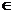Does not belong to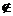For Every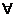There exists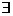Such that :

Set of Natural numbers N = {1,2,3,4 …} =  { n: nnatural numbers}

Set of whole numbers W = {0,1,2,3,….} = {n: n =0, and  n{N}}

2.15 Example1:

S = {2, 4, 8,16….} = { Numbers raised to the powers of 2 starting with 2} = {2m ;where   m is any natural number >1}

Let us do addition, multiplication, exponentiation operations on this set S. and we observe

1. The sum of any two numbers of S does not belong to S (ex; 6(=2+4),10(=2+8),12(=4+8) do not belong to S)

2.  The product of any two numbers of S belongs to the set S. Why?

(If  2m and 2n are  two  numbers then  the product is2m+n [= (2m )*(2n)] which belongs to S)

3.  The exponentiation of any 2 numbers of S belongs to the set S . Why ?

(If  2m and 2n are  two  numbers then  the exponent   2mz  [=(2m )z where z =2n  belongs to S)

Observation:  The result of sum ‘operation’ on any element of S does not belong to S. But the result of   multiplication and exponentiation ‘operations’  on any element of  S belongs to S

Definition:

1.If a, bA and the result of ‘operation’ on a, bA,  then we say that   A satisfies closure property w.r.t ‘operation’ ( w.r.t  is short form for with respect to)

2. If a, bA and c = a‘operation’bA, then we say that ‘operation’ is a Binary opeartion. By convention this ‘operation’ is denoted by ‘*’(not to be confused with multiplication)and

a ‘operation’ b is read as ‘a star b’.

In the case of example 1, we note that S does not satisfy closure property w.r.t addition and hence the addition ‘operation’ is not a Binary operation on S.

But multiplication and exponentiation ‘operations’ satisfy closure property and these two ‘operations’ are binary operations on S

Examples:

 No. Set Star () Observations Conclusion Reasoning 1 N = {1,2,3; Natural Numbers} sum,a,bN, a+bN N Satisfies Closure property w.r.t + Sum of 2 natural numbers is a natural number 2 N = {1,2,3; Natural Numbers} product,a,bN, a* bN N Satisfies Closure property w.r.t * Product  of 2 natural numbers is a natural number 3 A = {1,3,5: Odd numbers} sum,a,bN, a+bN A does not satisfy closure property w.r.t + Sum of 2 odd number is an even number 4 B = {1,3,5: Odd numbers} product,a,bN, a*bN B satisfies closure property w.r.t * Product  of 2 odd number is an odd number 5 Z =(0,-1,1,2,-2:  Integers) Average,a,bZ, ab=(a+b)/2Z Z does not satisfy closure property w.r.t  the ‘operation: Average’ Operation 0 star 1 = (0+1)/2 which is a fraction and not an integer 6 Q = (p/q, where p,qZ and q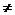0 Division,a,bQ, a/bQ, though  0Q Q does not Satisfy closure property w.r.t  the ‘operation: /’ Division of rational number by 0 is undefined ( Though  0Q, 1/0Q)

Relationship between Closure property and Binary operation:

If  any set satisfies the closure property w.r.t an operation then that operation is a binary operation and conversely if an operation on a set is binary operation then the set satisfies closure property w. r. t that operation.

Definition:

The ‘algebraic structure’ is a pattern such that the non empty set S and the ‘operation(*)’  on S  is a binary operation. The algebraic structure is denoted by the expression (S,*)

In the examples listed above we can see that  (N,+),(N,*),(B,*) are all  Algebraic structures and (A,+),(Z, average), (Q,/) are not Algebraic structures.

2.15 Summary of learning

 No Points to remember 1 If a, bA and the result of ‘operation’ on a, bA,  then we say that   A satisfies closure property w.r.t ‘operation’ 2 If  any set satisfies the closure property w.r.t an operation then that operation is a binary operation and conversely if an operation on a set is binary operation then the set satisfies closure property w. r. t that operation. 3 The Algebraic structure is a pattern such that the non empty set S and the ‘operation(*)’  on S  is a binary operation and  is denoted by the expression (S,*)